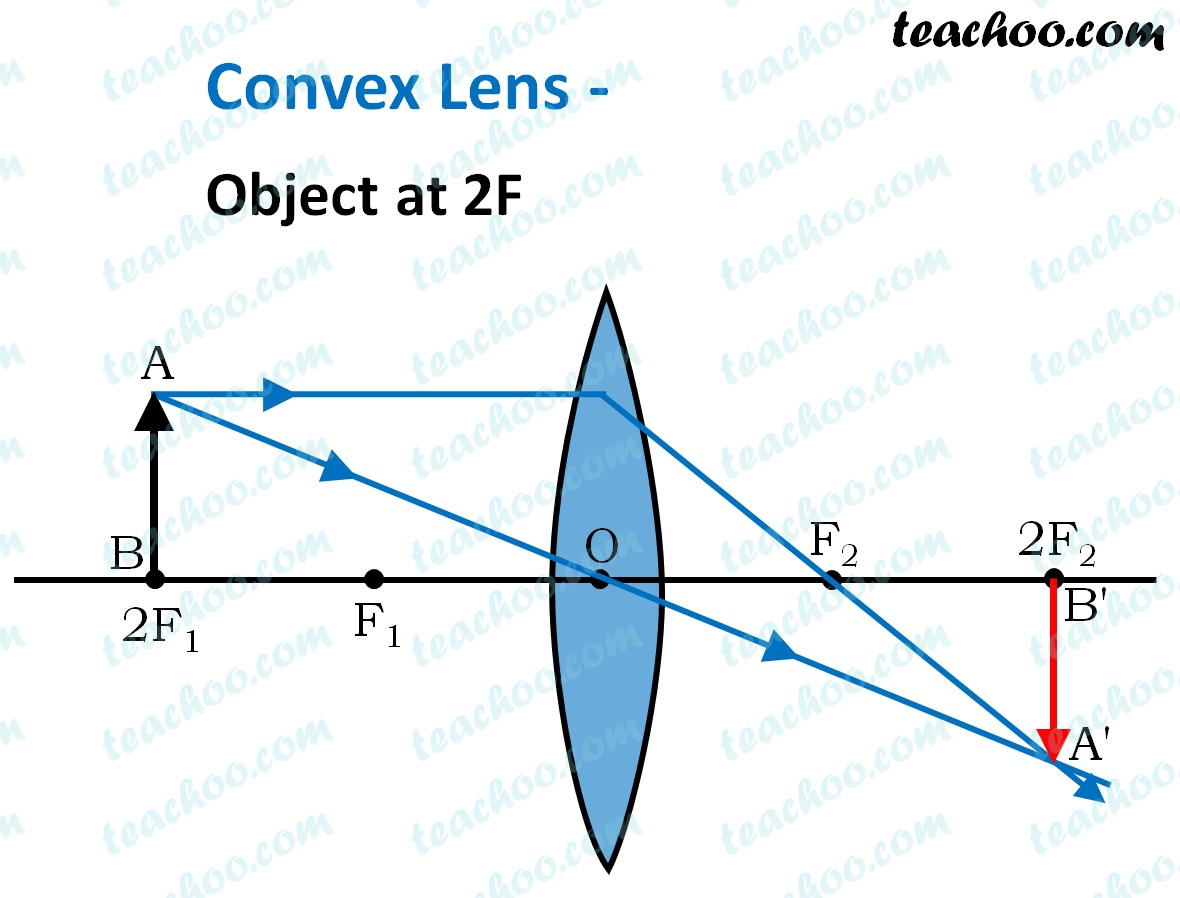Case Based Questions (MCQ)

Class 10
Chapter 10 Class 10 - Light - Reflection and Refraction

## A student focussed the image of a candle flame  on a white screen by placing the flame at various  distances from a convex lens. He noted his  observations as:

 S. No. Distance of flame  from the lens (cm) Distance of the screen  from the lens (cm) (i) 60 20 (ii) 40 24 (iii) 30 30 (iv) 24 40 (v) 15 70

### (iv) 60cm

We know that in a Convex lens

• If an object is kept at 2F , it will form at 2F

From table, we can see that

• Distance of image and Distance of object i s the same at 30 cm
• Thus, 2F = 30 cm
• So, Focal length = F = 15 cm

So, the correct answer is (i) 15 cm

### (iv) Object is kept at optical centre

 S. No. Distance of flame  from the lens (cm) Distance of the screen  from the lens (cm) (i) 60 20 (ii) 40 24 (iii) 30 30 (iv) 24 40 (v) 15 70

We check all observations one-by-one, for a convex lens of focal length 15 cm

• For observation (i) - Object is kept beyond 2F , so distance of image will be between F and 2F, i.e. between 15 and 30 cm . Since, it is 20 cm, this observation is correct

• For observation (ii) - Object is kept beyond 2F , so distance of image will be between F and 2F, i.e. between 15 and 30 cm . Since, it is 24 cm, this observation is correct

• For observation (iii) - Object is kept at 2F , so distance of image will also be 2F i.e. image distance is 30 cm . Since, it is 30 cm, this observation is correct

• For observation (iv) - Object is kept between F and 2F , so distance of image will be beyond 2F i.e. image distance is beyond 30 cm . Since, it is 40 cm, this observation is correct

• For observation (v) - Object is kept at F (i.e. 15 cm) , so distance of image will be at infinity i.e. image distance cannot be calculated . Since, it is 70 cm, this observation is incorrect

Now, in our options

• In Observation (v), image should be formed at infinity and not at 70 cm. So, this is not correct

So, the correct option is (iii) - Image should form at infinity

### (iv) In (i) caseFor a convex lens

• If object is kept at 2F , size of image and object is same
• Here, 2F = 30 cm
• So, in option (iii) , size of image and object is same

So, the correct answer is (iii)

### (iv) Size of the image increases slightly

In a convex lens

• As we move from infinity to Optical center
• First, image size is a point (object at Infinity)
• Then, image becomes bigger (object beyond 2F) until i t becomes the size of the objec t (object at 2F)
• Then image becomes bigger than the object as we move from 2F to F and then from F to O

Hence, size of image increases slightly.

So, the correct answer is (iv)

### (iv) It forms a real,inverted and enlarged image.

In a convex lens

• Real, inverted and diminished image is formed when object is kept beyond 2F.
So, option (i) is true

• Virtual, erect and enlarged image is formed when object is kept between F & O.
So, option (ii) is true

• Virtual, erect and diminished image cannot be formed.
So, option (iii) is false

• Real, inverted and enlarged image is formed when object is kept between F & 2F.
So, option (iv) is true

So, the correct answer is (iii)

Get live Maths 1-on-1 Classs - Class 6 to 12